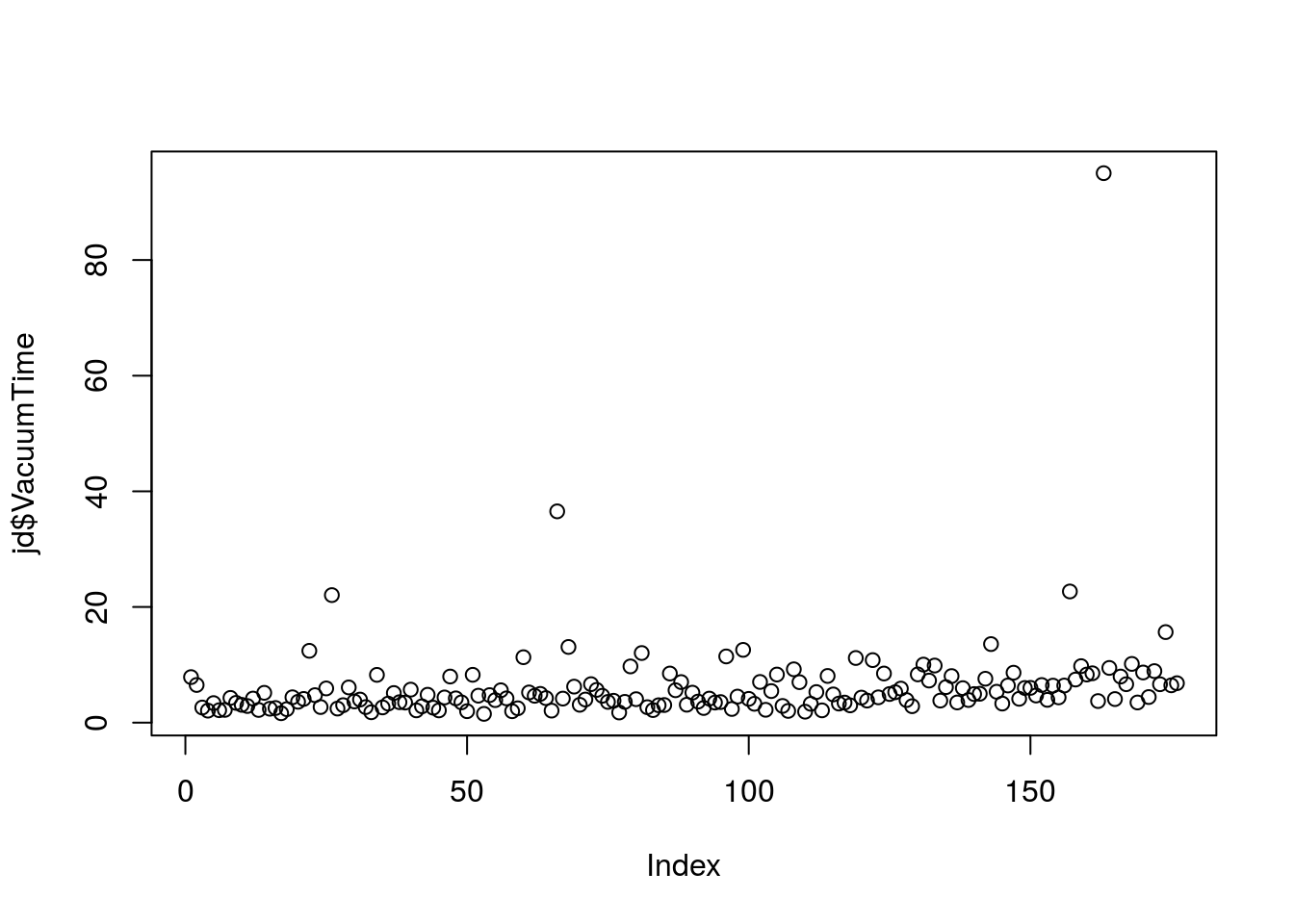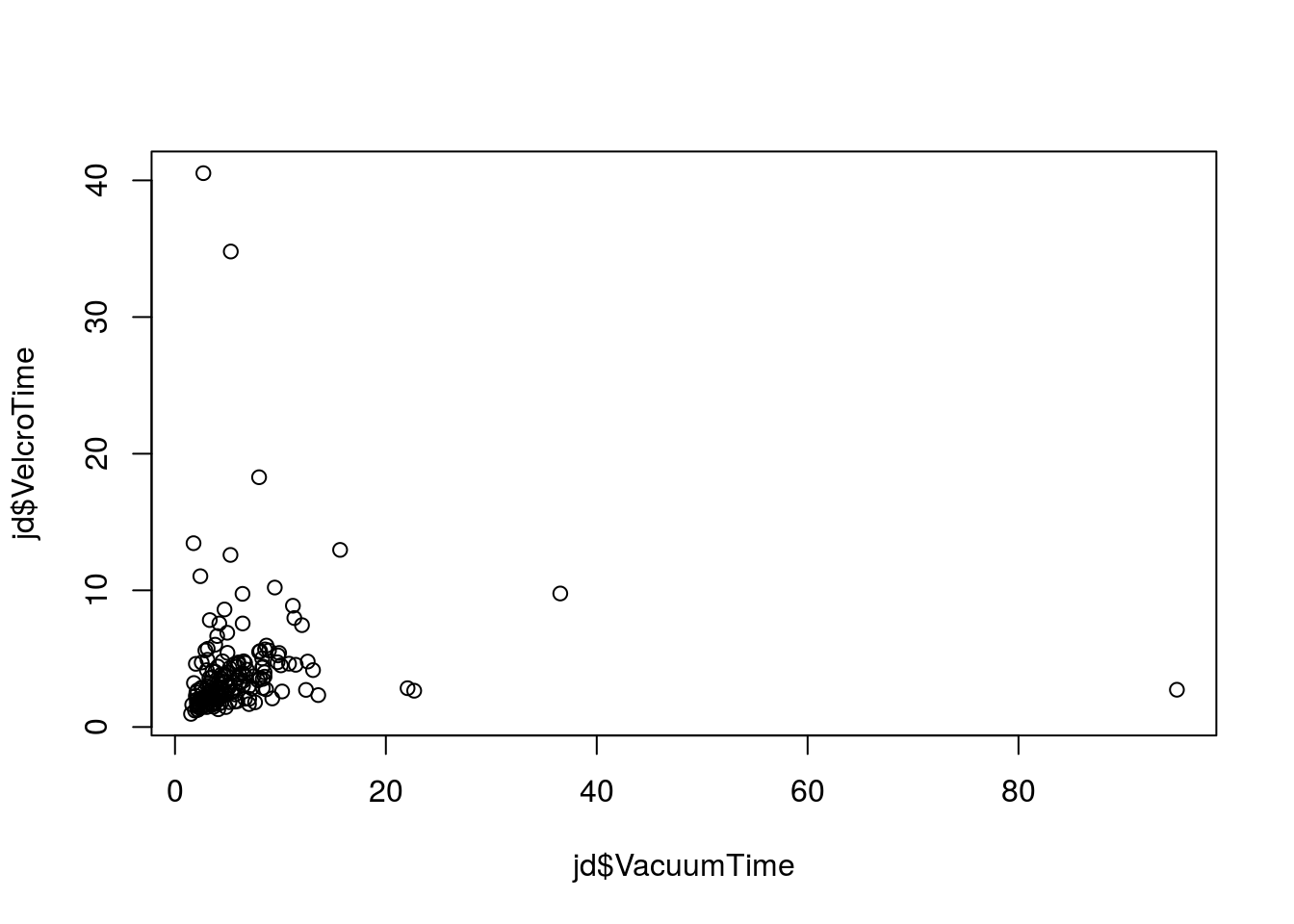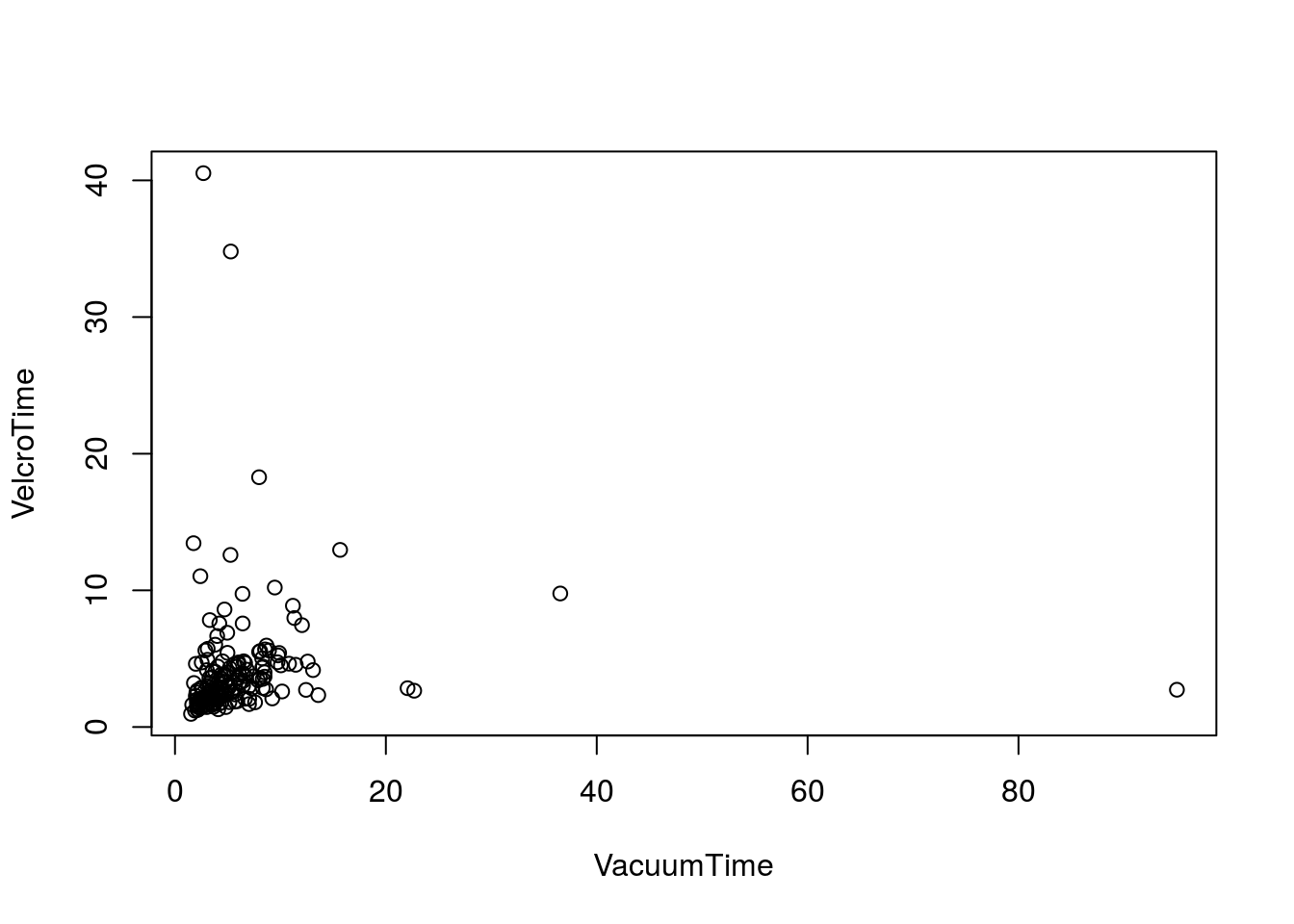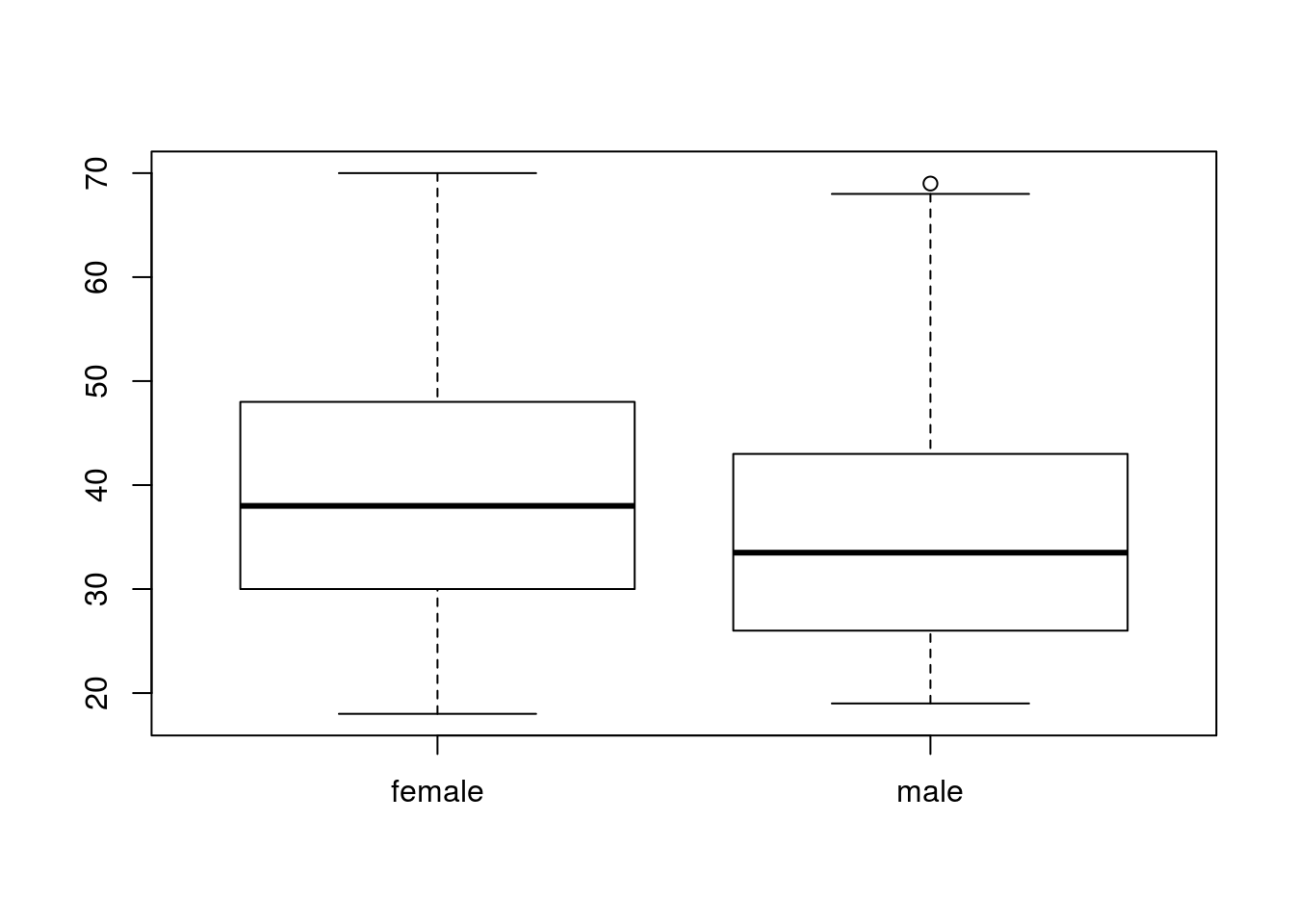For the last exercise I prepared a simple table for your to work with (codons.txt) that would be very easy to load into an R data frame with read.table. It was already formatted to work with the defaults and had a very simple structure. When working with data in-the-wild things are rarely quite so easy. You’ll often need to read data in from binary or proprietary formats and tidy up the input before you can start working with the data in R. For many projects this data wrangling phase is actually the hardest part. In this first case study, we’ll walk through importing and tidying an example data set originating in an Excel document.

Start the exercise

To update your project with the data and .rmd file for this exercise, run:

bio297::start()

To check your progress as you work through this exercise you can compile the document using the “Knit HTML” button on the R Studio tool bar.

Mechanical Turk survey

Mechanical Turk (or “mturk”) is an Amazon platform for recruiting large numbers of humans to do simple tasks that algorithms aren’t good at doing. The Johnson Lab also uses this platform to recruit large numbers of human participants to studies of human cognition. Today we’ll be working with an example data set that they’ve provided.

Download the file johnsonlab.xlsx from the data/ subfolder and take a quick look at it in Excel.

Reading from an Excel file

A number of R packages designed to read data from Excel into an R data frame have been released over the years. The most recent (and best in my opinion) is the readxl package.

I’ve already installed readxl for you, but if I hadn’t you could install this package with:

To load the functions in readxl into our current session, we’ll use library:

Packages and help pages

The best place to start with a new package is to take a look at the list of functions it exports. In RStudio, click on the “Packages” tab and click the link for the readxl package.

At the console you can use:

##  "excel_sheets" "read_excel"

This package is pretty simple. It exports just two functions: excel_sheets and read_excel. Read through the help pages for both and make sure you are able to parse the instructions.

The read_excel function seems simple enough! Let’s use it to load data from the first sheet:

Why didn’t we have to specify a sheet argument above?

Getting the lay of the land

Take a look at the data frame using the RStudio viewer. You can click on it in the “Data” section of the “Environment” tab or if you’d rather type than click:

View(jd)

It’s clear that this data has come to us by way of a stats lab; there are several things that have done right which are going to make our job easier:

• The data is tidy: each variable has a column, each observation a row
• Variable names are formatted consistently
• Variable names start with letters and have no spaces

If any of these things weren’t true we’d have some work to do to clean them up. Because column headings all need to be valid R variable names on data.frame’s they can’t start with numbers or have spaces in them. R will coerce invalid names to valid names automatically but the results will be ugly and hard to read.

These data are also a nice mix of variable types. We have:

• Continuous data: VacuumTime
• Discrete data: VacuumUnderstanding
• Categorical data: Gender

In R, numeric vectors are a good fit for modeling either continuous or discrete values. Let’s verify that read_excel imported these columns as numerics using the class function:

class(jd$VacuumTime) ##  "numeric" class(jd$VacuumUnderstanding)
##  "numeric"

Looking good. If these numbers had been surrounded by “” in Excel they could have imported as a character vector instead.

Now let’s check on a categorical variable:

class(jd$Gender) ##  "numeric" What happened here? Why? We’ll want to clean this up before throwing our data into summary or plotting functions. The facts about Factors R has a special type of vector designed to model categorical values: the factor. For folks new to R, the difference between character vectors and factors can be a bit confusing, since both hold text (and factors are quite unique to the R data model). However, it’s important to keep them straight and be sure you’re using the right tool for your data. In general: • Use character to hold arbitrary text: for example codon sequences • Use factor to hold true categorical variables (Gender) with defined levels (male, female). You can create factors with the factor or as.factor functions. The simplest usage is to pass in a character vector: gender <- factor( c("male", "female") ) gender ##  male female ## Levels: female male We’ll choose this order for the levels to be consistent with “variable key” we were given in the original Excel file. You can see that factors look different than character vectors when they’re printed out. First, there are no “”’s around the text. Second, there’s a “Levels:” summary. If you just want the levels of a factor returned as a character vector you can use the levels function: levels(gender) ##  "female" "male" You can index factors just like any other type of vector: gender[ c(1, 1, 2, 2, 1) ] ##  male male female female male ## Levels: female male Are you starting to feel like an indexing ninja yet? Make sure you understand what happened there before you go on! Using factors to model categorical data R stats and plotting functions are usually smart about factors and will apply logic that is appropriate to categorical data when given factors as a variable. Because of this, it’s a good idea to make sure all of the categorical columns on your data frames are converted to factors before you start exploring the data. Let’s change the Gender column so that it’s a factor rather than a numeric. Because we’ve setup our gender factor so that 1 = male and 2 = female we can leverage the fact that our$Gender column is already numeric.

We can replace the current numeric column with a factor:

jd$Gender <- gender[ jd$Gender ]

Check to make sure that worked and verify that you understand why it did!

Your turn: turn the other variables that should be categorical into factors. Do this part of the exercise after class.

# Enter your code here!

Summary statistics

Now that we’ve tidied up our data frame we’re ready to start exploring it. R provides a handy summary function that provides summary information that is appropriate for the input data type.

You can call summary on an entire data frame:

summary(jd)

Or just one vector:

summary(jd$VacuumTime) ## Min. 1st Qu. Median Mean 3rd Qu. Max. ## 1.519 3.286 4.384 6.092 6.696 95.030 summary(jd$Gender)
## female   male
##    118     58

Notice how the statistics that are calculated are different for different types of vectors. The summary function is a little bit magical in this regard.

Basic plotting

Just like summary, the plotting functions like plot and boxplot are also a little bit magical in R.

We can plot one numeric column:

plot(jd$VacuumTime)Or make a scatter plot with two: plot(jd$VacuumTime, jd\$VelcroTime)Formula syntax

In data analysis, you’re often exploring how variables interact with a particular model in mind. For example, if we were to use that last scatter plot to do a regression analysis our model would have been: does VelcroTime vary as a function of VacuumTime?

Exploring models is such a common task that R has a neat little syntax for expressing relationships between variables. The simplest formula syntax is DEPENDANT ~ INDEPENDANT.

So that last scatter plot in formula syntax would be:

plot(VelcroTime ~ VacuumTime, data = jd)Notice the magic there? We used the variables VelcroTime and VacumeTime in our formula and then told R where to find them with the data argument. Also, check out what happened to the x- and y-axis labels.

The plot function works well when comparing two quantitative variables. If you want to compare a quantitative and categorical variable boxplot is a good choice:

boxplot(Age ~ Gender, data = jd)